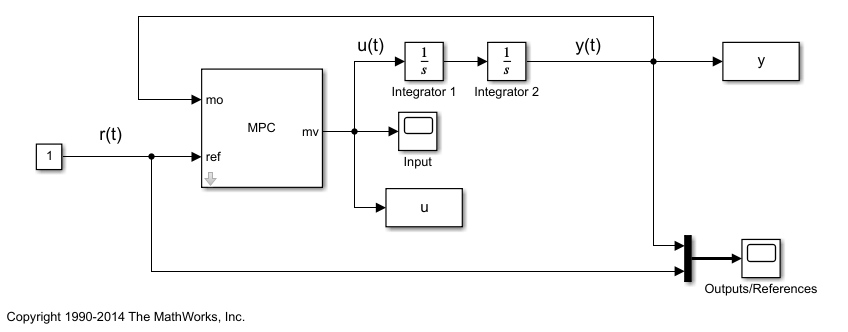# Use the GPU to Simulate an MPC Controller in Simulink

This example shows how to generate CUDA® code and use the GPU to compute optimal MPC moves in Simulink®.

### Create Plant Model and Design MPC Controller

Use a double integrator as a plant.

`plant = tf(1,[1 0 0]);`

Create an MPC object for the plant with a sampling time of 0.1 seconds, and prediction and control horizon of 10 and 3 steps, respectively.

`mpcobj = mpc(plant, 0.1, 10, 3);`
```-->The "Weights.ManipulatedVariables" property of "mpc" object is empty. Assuming default 0.00000. -->The "Weights.ManipulatedVariablesRate" property of "mpc" object is empty. Assuming default 0.10000. -->The "Weights.OutputVariables" property of "mpc" object is empty. Assuming default 1.00000. ```

Limit the manipulated variable between –1 and 1.

`mpcobj.MV = struct('Min',-1,'Max',1); `

### Control the Plant Model in Simulink

Create a Simulink closed loop simulation using the MPC Controller block, with the `mpcobj` object passed as a parameter, to control the double integrator plant. For this example, open the pre-existing `gpudemo` Simulink model.

`open_system('gpudemo')`Open the Configuration Parameters dialog box by clicking Model Settings. Then, in the Code Generation section, select Generate GPU code.You can now run the model by clicking Run or by using the MATLAB® command `sim`. Before running the simulation the model will generate CUDA code from the Simulink model and compile it to obtain a MEX executable. When the model is simulated, this file is called and the simulation is performed on the GPU.

`sim('gpudemo')`
```-->Converting the "Model.Plant" property of "mpc" object to state-space. -->Converting model to discrete time. Assuming no disturbance added to measured output channel #1. -->The "Model.Noise" property of the "mpc" object is empty. Assuming white noise on each measured output channel. ```

After the simulation, the plots of the two scopes show that the manipulated variable does not exceed the limit and the plant output tracks the reference signal after approximately 3 seconds.Here, the entire Simulink model is executed on the GPU. To deploy only the MPC block on the GPU, you can create a model having only the MPC block inside. Typically in embedded control modules, the deployed model contains the controller block plus a few interface blocks for input/output signals.